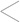Abreviations used
0 = Value to be entered
R = Final result
1 ct = 41.666 ml

Alloy = Alloy
Au 24 ct = 24 Carat Gold
MT = Metal (g)
MTD = Metal Density
OD = Object Density
F = Higher fineness (ml)
f = Lower fineness (ml)
W = Weight (g)
IW = Ingot Weight (g)
OW = Object Weight (g)
IF = Ingot Fineness (ml)
Carat (ct)
Millesimal
fineness (ml)
Grams (g)
Convert carats to millesimal fineness
R ml
Convert millesimal fineness to carats
R ct
Given the weight and fineness of an ingot, determine the amount of 14 carat gold it contains
R g
Determine the final weight of an alloyed metal
R g alloyed gold
Increase the fineness of a given alloy
0
R g
Decrease the fineness of a given alloy
R g
Given an object of a certain metal and its density, determine its weight in gold or silver
R g